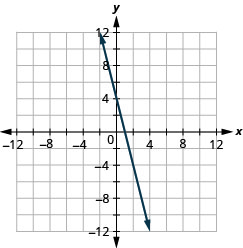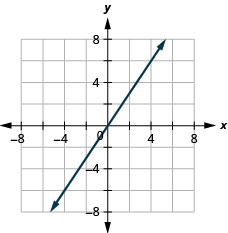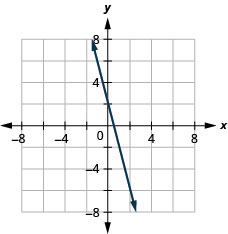# 3.2E：练习

•• OpenStax
• OpenStax
$$\newcommand{\vecs}{\overset { \rightharpoonup} {\mathbf{#1}} }$$ $$\newcommand{\vecd}{\overset{-\!-\!\rightharpoonup}{\vphantom{a}\smash {#1}}}$$$$\newcommand{\id}{\mathrm{id}}$$ $$\newcommand{\Span}{\mathrm{span}}$$ $$\newcommand{\kernel}{\mathrm{null}\,}$$ $$\newcommand{\range}{\mathrm{range}\,}$$ $$\newcommand{\RealPart}{\mathrm{Re}}$$ $$\newcommand{\ImaginaryPart}{\mathrm{Im}}$$ $$\newcommand{\Argument}{\mathrm{Arg}}$$ $$\newcommand{\norm}{\| #1 \|}$$ $$\newcommand{\inner}{\langle #1, #2 \rangle}$$ $$\newcommand{\Span}{\mathrm{span}}$$ $$\newcommand{\id}{\mathrm{id}}$$ $$\newcommand{\Span}{\mathrm{span}}$$ $$\newcommand{\kernel}{\mathrm{null}\,}$$ $$\newcommand{\range}{\mathrm{range}\,}$$ $$\newcommand{\RealPart}{\mathrm{Re}}$$ $$\newcommand{\ImaginaryPart}{\mathrm{Im}}$$ $$\newcommand{\Argument}{\mathrm{Arg}}$$ $$\newcommand{\norm}{\| #1 \|}$$ $$\newcommand{\inner}{\langle #1, #2 \rangle}$$ $$\newcommand{\Span}{\mathrm{span}}$$$$\newcommand{\AA}{\unicode[.8,0]{x212B}}$$

## 练习成就完美

1. a.$$(−4,2)$$ b.$$(−1,−2)$$ c.$$(3,−5)$$ d.$$(−3,0)$$
e.$$(53,2)$$2. a.$$(−2,−3)$$ b.$$(3,−3)$$ c.$$(−4,1)$$ d.$$(4,−1)$$
e.$$(32,1)$$

3. a.$$(3,−1)$$ b.$$(−3,1)$$ c.$$(−2,0)$$ d.$$(−4,−3)$$
e.$$(1,145)$$4. a.$$(−1,1)$$ b.$$(−2,−1)$$ c.$$(2,0)$$ d.$$(1,−4)$$
e.$$(3,72)$$

a. 有序对是方程的解吗？ b. 点在直线上吗？

5。 $$y=x+2$$;

A:$$(0,2)$$; B:$$(1,2)$$; C:$$(−1,1)$$; D:$$(−3,−1)$$a. A：是，B：否，C：是，D：是 b。A：是，B：否，C：是，D：是

6。 $$y=x−4$$;

A:$$(0,−4)$$; B:$$(3,−1)$$; C:$$(2,2)$$; D:$$(1,−5)$$7。 $$y=12x−3$$;
A:$$(0,−3)$$; B:$$(2,−2)$$; C:$$(−2,−4)$$; D:$$(4,1)$$a. A：是，B：是，C：是，D：否 b。A：是，B：是，C：是，D：否

8。 $$y=13x+2$$;
A:$$(0,2)$$; B:$$(3,3)$$; C:$$(−3,2)$$; D:$$(−6,0)$$9。 $$y=x+2$$10。 $$y=x−3$$

11。 $$y=3x−1$$12。 $$y=−2x+2$$

13。 $$y=−x−3$$14。 $$y=−x−2$$

15。 $$y=2x$$16。 $$y=−2x$$

17。 $$y=12x+2$$18。 $$y=13x−1$$

19。 $$y=43x−5$$20。 $$y=32x−3$$

21。 $$y=−25x+1$$22。 $$y=−45x−1$$

23。 $$y=−32x+2$$24。 $$y=−53x+4$$

25. a.$$x=4$$ b.$$y=3$$b。26. a.$$x=3$$ b.$$y=1$$

27. a.$$x=−2$$ b.$$y=−5$$b。28. a.$$x=−5$$ b.$$y=−2$$

29。 $$y=2x$$$$y=2$$30。 $$y=5x$$$$y=5$$

31。 $$y=−12x$$$$y=−12$$32。 $$y=−13x$$$$y=−13$$

33。$$(3,0),(0,3)$$

34。35。$$(5,0),(0,−5)$$

36。37。 $$x−y=5$$

$$x$$-int:$$(5,0)$$,$$y$$-int:$$(0,−5)$$

38。 $$x−y=−4$$

39。 $$3x+y=6$$

$$x$$-int:$$(2,0)$$,$$y$$-int:$$(0,6)$$

40。 $$x−2y=8$$

41。 $$4x−y=8$$

$$x$$-int:$$(2,0)$$,$$y$$-int:$$(0,−8)$$

42。 $$5x−y=5$$

43。 $$2x+5y=10$$

$$x$$-int:$$(5,0)$$,$$y$$-int:$$(0,2)$$

44。 $$3x−2y=12$$

45。 $$−x+4y=8$$46。 $$x+2y=4$$

47。 $$x+y=−3$$48。 $$x−y=−4$$

49。 $$4x+y=4$$50。 $$3x+y=3$$

51。 $$3x−y=−6$$52。 $$2x−y=−8$$

53。 $$2x+4y=12$$54。 $$3x−2y=6$$

55。 $$2x−5y=−20$$56。 $$3x−4y=−12$$

57。 $$y=−2x$$58。 $$y=5x$$

59。 $$y=x$$60。 $$y=−x$$

61。 $$y=32x$$62。 $$y=−23x$$

63。 $$y=−12x+3$$64。 $$y=14x−2$$

65。 $$4x+y=2$$66。 $$5x+2y=10$$

67。 $$y=−1$$68。 $$x=3$$

## 写作练习

69。 解释如何选择三个 x 值来制作一个表格来绘制线条图$$y=15x−2$$

70。 垂直线和水平线的方程有什么区别？

71。 你更喜欢使用绘制点的方法还是使用截距的方法来绘制方程式$$4x+y=−4$$？ 为什么？

72。 你更喜欢使用绘制点的方法还是使用截距的方法来绘制方程式$$y=23x−2$$？ 为什么？

## 自检

a. 完成练习后，使用此清单评估您对本节目标的掌握程度。b. 如果您的大部分支票是：